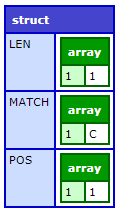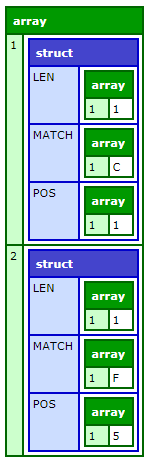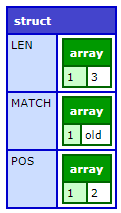# REFind

### Description

Uses a regular expression (RE) to search a string for a pattern. The search is case sensitive. For more information on regular expressions, including escape sequences, anchors, and modifiers, see Using Regular Expressions in Functions in the Developing ColdFusion Applications.

### Returns

Depends on the value of the returnsubexpressions__parameter:

• If  returnsubexpressions = "False":
• The position in the string where the match begins
• 0, if the regular expression is not matched in the string
• If returnsubexpressions = "True": a structure that contains two arrays, len and pos. The array elements are as follows:
• If the regular expression is found in the string, the first element of the len and pos arrays contains the length and position, respectively, of the first match of the entire regular expression.
• If the regular expression contains parentheses that group subexpressions, each subsequent array element contains the length and position, respectively, of the first occurrence of each group.
• If the regular expression is not found in the string, the first element of the  len  and pos arrays contains 0.

### History

• ColdFusion (2018 release) Update 5: Added the flag useJavaAsRegexEngine to Application.cfc. Enable this flag to use Java Regex as the default regex engine. For more information, see Application variables. For information on using Regular Expression using the flag, see Using Regular Expressions.
• ColdFusion (2016 release): Added the scope parameter.

### Function syntax

`REFind(reg_expression, string [, start, returnsubexpressions, scope ] )`

### Parameters

Parameter

Description

reg_expression

Regular expression for which to search. Case sensitive.

string

A string, or a variable that contains one, in which to search.

start

Optional. A positive integer, or a variable that contains one. Position in the string at which to start search. The default value is 1.

returnsubexpressions

Optional. Boolean. Whether to return substrings of reg_expression, in arrays named len and pos:

• True: if the regular expression is found, the first array element contains the length and position, respectively, of the first match. If the regular expression contains parentheses that group subexpressions, each subsequent array element contains the length and position, respectively, of the first occurrence of each group. If the regular expression is not found, the arrays each contain one element with the value 0.
• False: the function returns the position in the string where the match begins. Default.

scope

Optional

• one: returns the first value that matches the regex.
• all: returns all values that matches the regex.

### Usage

This function finds the first occurrence of a regular expression in a string. To find the second and subsequent instances of the expression or of subexpressions in it, you call this function more than once, each time with a different start position. To determine the next start position, use the returnsubexpressions__parameter, and add the value returned in the first element of the length array to the value in the first element of the position array.

### Examples

Examples using the scope parameter

```<cfscript>
// when start=1, returnSubexpressions=true, and scope="one"
writeDump(REFind("[A-Z]+", "ColdFusion 123!",1,true,"one"));
</cfscript>```

Output```<cfscript>
// when start=1, returnSubexpressions=true, and scope="all"
writeDump(REFind("[A-Z]+", "ColdFusion 123!",1,true,"all"));
</cfscript>```

Output```<cfscript>
// when start=1, returnSubexpressions=true, and scope="one"
writeDump(REFind("[a-z]+", "ColdFusion 123!",1,true,"one"));
</cfscript>```

Output```<cfscript>
// when start=1, returnSubexpressions=true, and scope="all"
writeDump(REFind("[a-z]+", "ColdFusion 123!",1,true,"all"));
</cfscript>```

OutputBei Ihrem Konto anmelden